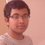# Interesting Fibonacci Identity

$\color{#D61F06}{\large \sum^{N}_{n=1}F_n \,=\, F_{N+2} -1}$.

I was trying to solve this problem. Whilst solving this question, I figured out a very interesting formula, which is as shown above.

I don't know whether this formula has already been discovered or not, but, anyways here is the proof. $\huge \color{#3D99F6}{\text{Proof :-}}$ By $\color{#20A900}{\text{Binet's Fibonacci Formula}}$, we know that : $\color{#D61F06}{\large F_n\,=\,\frac{(1+\sqrt5)^{n}\,-\,(1-\sqrt5)^{n}}{2^{n} \cdot \sqrt5 \cdot }}$, where $n\,\epsilon\,\mathbb{N}$. Say $S \,=\, \sum^{N}_{n=1}F_n$. So, $\large S \,=\, \sum^{N}_{n=1}\frac{(1+\sqrt5)^{n}\,-\,(1-\sqrt5)^{n} }{2^{n} \cdot \sqrt5}$ $\implies S \,=\, \frac{1}{\sqrt5} \left( \frac{\frac{1+\sqrt5}{2}\,\cdot \, \left ( 1 \,-\, \left ( \frac{1+\sqrt5}{2} \right )^{N} \right )}{1 - \frac{1+\sqrt5}{2}} \right) \,-\, \frac{1}{\sqrt5} \left( \frac{\frac{1-\sqrt5}{2}\,\cdot \, \left ( 1 \,-\, \left ( \frac{1-\sqrt5}{2} \right )^{N} \right )}{1 - \frac{1-\sqrt5}{2}} \right)$. This on simplification yields :- $S \,=\, \frac{1}{\sqrt5} \left( \frac{(1+\sqrt{5})^{N+2}-(1-\sqrt{5})^{N+2}-2^{N+2}\sqrt{5}}{2^{N+2}} \right)$ $S \,=\, \left(\frac{(1+\sqrt{5})^{N+2} - (1-\sqrt{5})^{N+2}}{2^{N+2} \cdot \sqrt{5}} \right) \,-\, 1$ $\large \boxed{\color{#20A900}{\sum^{N}_{n=1}F_n \,=\, F_{N+2} -1}}$ Check this formula for this question.Note by Aditya Sky
5 years ago

This discussion board is a place to discuss our Daily Challenges and the math and science related to those challenges. Explanations are more than just a solution — they should explain the steps and thinking strategies that you used to obtain the solution. Comments should further the discussion of math and science.

When posting on Brilliant:

• Use the emojis to react to an explanation, whether you're congratulating a job well done , or just really confused .
• Ask specific questions about the challenge or the steps in somebody's explanation. Well-posed questions can add a lot to the discussion, but posting "I don't understand!" doesn't help anyone.
• Try to contribute something new to the discussion, whether it is an extension, generalization or other idea related to the challenge.
• Stay on topic — we're all here to learn more about math and science, not to hear about your favorite get-rich-quick scheme or current world events.

MarkdownAppears as
*italics* or _italics_ italics
**bold** or __bold__ bold
- bulleted- list
• bulleted
• list
1. numbered2. list
1. numbered
2. list
Note: you must add a full line of space before and after lists for them to show up correctly
paragraph 1paragraph 2

paragraph 1

paragraph 2

[example link](https://brilliant.org)example link
> This is a quote
This is a quote
    # I indented these lines
# 4 spaces, and now they show
# up as a code block.

print "hello world"
# I indented these lines
# 4 spaces, and now they show
# up as a code block.

print "hello world"
MathAppears as
Remember to wrap math in $$ ... $$ or $ ... $ to ensure proper formatting.
2 \times 3 $2 \times 3$
2^{34} $2^{34}$
a_{i-1} $a_{i-1}$
\frac{2}{3} $\frac{2}{3}$
\sqrt{2} $\sqrt{2}$
\sum_{i=1}^3 $\sum_{i=1}^3$
\sin \theta $\sin \theta$
\boxed{123} $\boxed{123}$

## Comments

Sort by:

Top Newest

There is also a "one-line" induction proof.

Staff - 5 years ago

Log in to reply

There is a much simpler way to derive this formula.

Hint: Telescoping.

Log in to reply

×

Problem Loading...

Note Loading...

Set Loading...